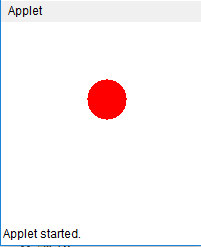# Java Applet program to show the animation of a bouncing ball

Here, we are implementing a java program that will display an animation of bouncing ball. This is an example of java applet program.
Submitted by Chandra Shekhar, on January 11, 2018

Given coordinates of the box, radius of the circle and color using these elements we have to make an animation of a bouncing ball using Java applet program.

### Applet program to show animation of bouncing ball

```// This java applet program will show
// the bouncing balls

package Applet;

import java.applet.*;
import java.awt.*;

public class BouncingBall extends Applet implements Runnable {
// x,y coordinates and radius of the circle.
int x = 150, y = 50, r = 20;
int dx = 11, dy = 7;

boolean stopFlag;

public void start() {
stopFlag = false;
t.start();
}

// Draw cicle from its present position.
public void paint(Graphics g) {
g.setColor(Color.red);
g.fillOval(x - r, y - r, r * 2, r * 2);
}

// function to move the image.
public void run() {
while (true) {
if (stopFlag)
break;
// Bounce if we've hit an edge.
if ((x - r + dx < 0) || (x + r + dx > bounds().width)) dx = -dx;
if ((y - r + dy < 0) || (y + r + dy > bounds().height)) dy = -dy;
// Move the circle.
x += dx;
y += dy;

try {
} catch (Exception e) {
System.out.println(e);
};
// print circle again n again.
repaint();
}
}

// function to stop printing.
public void stop() {
stopFlag = true;
t = null;
}
}
```

OutputWhat's New

Top Interview Coding Problems/Challenges!

Languages: » C » C++ » C++ STL » Java » Data Structure » C#.Net » Android » Kotlin » SQL
Web Technologies: » PHP » Python » JavaScript » CSS » Ajax » Node.js » Web programming/HTML
Solved programs: » C » C++ » DS » Java » C#
Aptitude que. & ans.: » C » C++ » Java » DBMS
Interview que. & ans.: » C » Embedded C » Java » SEO » HR
CS Subjects: » CS Basics » O.S. » Networks » DBMS » Embedded Systems » Cloud Computing
» Machine learning » CS Organizations » Linux » DOS
More: » Articles » Puzzles » News/Updates